JEE  >  Solubility of liquids in liquids and Gases in Liquids

# Solubility of liquids in liquids and Gases in Liquids - Notes | Study Chemistry for JEE - JEE

 1 Crore+ students have signed up on EduRev. Have you?

SOLUBILITY OF LIQUIDS IN LIQUIDS
Water is known as a universal solvent as it dissolves almost every solute except for a few. Certain factors can influence the solubility of a substance. Solubility is the new bond formation between the solute molecules and solvent molecules.
In terms of quantity, solubility is the maximum concentration of solute that dissolves in a known concentration of solvent at a given temperature.
Based on the concentration of solute dissolves in a solvent, solutes are categorized into highly soluble, sparingly soluble or insoluble.

• If a concentration of 0.1 g or more of a solute can be dissolved in a 100ml solvent, it is said to be soluble.
• While a concentration below 0.1 g is dissolved in the solvent it is said to be sparingly soluble.

Thus, it is said that solubility is a quantitative expression and expressed by the unit gram/ liter (g/L).
Based on solubility, different types of solution can be obtained. A saturated solution is a solution where a given amount of solute is completely soluble in a solvent at given temperature.
On the other hand, a supersaturated solution is those where solute starts to salting out or precipitate after a particular concentration is dissolved at the same temperature.

SOLUBILITY OF GASES IN LIQUIDS

• Gas solubility in liquids deals with the concept of gas dissolving in a solvent.
• Let us first define solubility. For any substance, solubility is the maximum amount of solute that can be dissolved in a given solvent at a particular temperature.
• Now our concern is gas solubility in liquids. The gas solubility in liquids is greatly affected by temperature and pressure as well as the nature of the solute and the solvent.

The common examples are carbonated beverages, i.e., soft drinks, household cleaners containing aqueous solutions of ammonia, formalin-an aqueous solution of formaldehyde, etc.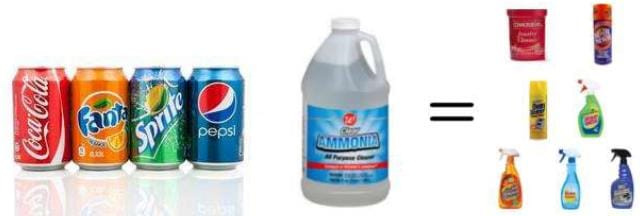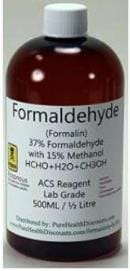The natural waters contain dissolved; O2 which is vital for the existence of aquatic life in sea, rivers and lakes.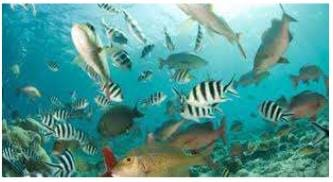There are many gases that readily dissolve in water, while there are gases that do not dissolve in water under normal conditions.
Oxygen is only sparingly soluble in water while HCl or ammonia readily dissolves in water.
The solubility of a gas in a liquid is expressed in terms of absorption coefficient. It is defined as the volume of the gas in mL that can be dissolved by 1 mL of a liquid solvent at the temperature of the experiment at one atmospheric pressure. The volume of the gas is measured at STP. Thus, if v is the volume of the gas dissolved, reduced to STP, V is the volume of the solvent and P is pressure of the gas in atmospheres, then the absorption
coefficient, a, is given by
α = v/VP

FACTORS AFFECTING SOLUBILITY
1. Nature of the gas and solvent: Generally, the gases which can be easily liquefied are more soluble in common solvents.
Example: CO2 is more soluble in water than oxygen or hydrogen.The gases which react with the solvent posses higher solubility. HCI and NH3 are highly soluble in water. Oxygen, nitrogen and carbon dioxide are much more soluble in ethyl alcohol than in water. at the same temperature and pressure.

2. Effect of Temperature: By changing the temperature we can increase the soluble property of a solute. Generally, water dissolves solutes at 20C or 100C. Sparingly soluble solid or liquid substances can be dissolved completely by increasing the temperature.
But in case of gaseous substance, temperature inversely influences solubility i.e. as the temperature increases gases expand and escapes from their solvent. Hence, Gas solubility in liquids is found to decrease with increase in temperature.
The gas molecules in a liquid are dissolved by the process of dissolution. During this process, heat is evolved. According to Le Chatelier’s Principle which states that when the equilibrium of a system is disturbed, the system readjusts itself in such way that the effect that has caused the change in equilibrium is countered.
So, as we know that dissolution is an exothermic process, the solubility should decrease with increase in temperature to validate Le Chatelier’s Principle.
Solubility of a gas in a liquid decreases with increase in temperature.
Dissolution of gas in liquid is considered similar to condensation and heat is evolved in this process. Since dissolution of gas in liquid is an exothermic process the increase in temperature resulting in decrease in solubility of gas in liquid.

This is the cause that aquatic animals are more comfortable in cold water rather than in warm water.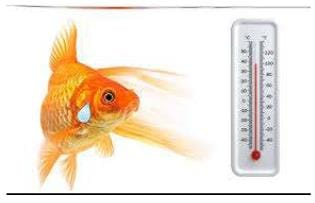3. Effect of Pressure: Gaseous substances are much influenced than solids and liquids by pressure. When the partial pressure of gas increases, the chance of its solubility is also increased.
A soda bottle is an example of where CO2 is bottled under high pressure.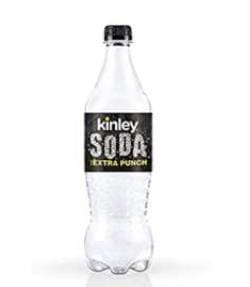It has been found that the gas solubility in liquids increases with increase in pressure.  To have a better understanding of the effect of pressure on gas solubility let us consider a system of a gas solution in a solvent in a closed container in a state of dynamic equilibrium. Now the solution is in equilibrium and hence the rate of gaseous molecules entering the solution is equal to the rate of gaseous molecules leaving the solution.
Now suppose we increase the pressure of the system by compressing the gas molecules present in the solution. As a result of an increase in pressure, the gases molecules will now be concentrated in a smaller volume. This will result in an increase in the number of gas molecules per unit volume available above the solution. Since the number of gas molecules presents above the solution has increased, the rate with which the gas molecules will be entering the solution will also increase. The end result is an increase in the number of gas molecules in the solution until a new equilibrium point is attained.
Thus the solubility of gases increases with increase in the pressure of a gas above the solution.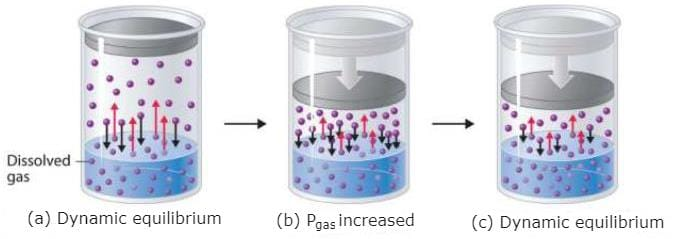The document Solubility of liquids in liquids and Gases in Liquids - Notes | Study Chemistry for JEE - JEE is a part of the JEE Course Chemistry for JEE.
All you need of JEE at this link: JEE

## Chemistry for JEE

322 videos|586 docs|368 tests

## Chemistry for JEE

322 videos|586 docs|368 tests

Track your progress, build streaks, highlight & save important lessons and more!

,

,

,

,

,

,

,

,

,

,

,

,

,

,

,

,

,

,

,

,

,

;